Monte Carlo方法的最优源项偏倚抽样密度函数

Monte Carlo粒子输运中的源项偏倚抽样方法可以减小方差、提高计算效率。该文通过建立一个多区域分权重数学投篮模型,模拟了输运过程中的源项信息,得到了源项偏倚抽样方差最小时的最佳抽样密度函数解析式。采用随机数值方法对模型进行了计算,验证了函数的正确性,并举一例实际的粒子输运模拟问题,表明最优偏倚抽样方法对减小方差的效果显著。该方法可作为一种普适的减方差技巧应用于Monte Carlo粒子输运中,可用于构造粒子源参数(如位置、发射方向等)的最佳偏倚密度函数,尤其在分层抽样时能给出方差最小的最优各层比例系数。

Optimal source biased sampling density function for the Monte Carlo method
Key Laboratory of Particle and Radiation Imaging of Ministry of Education, Department of Engineering Physics, Tsinghua University, Beijing 100084, China
Abstract

The source biased sampling method reduces the variance and improves the efficiency of Monte Carlo particle transport calculations. This paper gives a multi-region, multi-weight shooting model to simulate the source in transport processes. The density function gives the minimum variance for source biased sampling. The model is solved using a random numerical method to verify the correctness of the function. A particle transportation problem is then simulated to show the significant effect of the variance reduction. This method can be used as a general variance reduction technique in Monte Carlo particle transport analyses to construct the density function for biased source sampling for various particle source parameters, such as the transmission location and direction. It gives the best partition coefficient with the minimum variance in stratified sampling.

Keyword: Monte Carlo method; source biasing sampling; variance reduction; optimal bias density function; stratified sampling

Monte Carlo (MC)方法是一种随机实验方法,其基本原理是利用大量随机实验的平均结果来近似所要求解的问题的解。由于MC方法与所求解的问题维数无关,收敛速度可以预测,因此在粒子输运相关的物理研究中得到广泛应用[2,3]。在应用MC的过程中,为了得到较精确的模拟结果,统计方差要尽可能地小; 同时,为了提高计算效率,计算时间要尽可能地少,但通常研究以前者为研究重点,所研究的方法也称为“减方差技巧”[4,5,6]。当前,已经研究出了多种减方差技巧,如区域粒子分裂-轮盘赌、 Dxtran球[7,8]、 相关抽样[8,11]、自动重要抽样、指数变换[13,14,15]、权重窗、方向偏移等,这些技巧已经在MCNP、 FLUKA、 GEANT4等大型MC程序中得到实现[16,17,18,19,20,21]。在实际应用中,经常使用源偏倚抽样方法,通过改变源项的分布函数,对贡献大的区域多抽样,对贡献小的区域少抽样,再以纠偏权重使统计结果无偏,从而在计算时间基本相同的情况下实现减小方差的目的。本质上,源偏倚抽样属于重要抽样方法,已有多篇文献给出了重要抽样的最佳重要性函数[4,5],但是这些函数都是理论上的“零方差”表达式,在实际的模拟中很难直接应用。本文以投篮数学模型为基础,得到了源偏倚抽样条件下使方差最小的密度函数,它对于源抽样的各类参数(如位置、发射方向等)具有普适性,是可以应用于MC粒子输运的减方差技巧。

1 问题的提出

1.1 多区域投篮问题的概率论描述

$PY|X(y=1|x=A)=0.1,PY|X(y=1|x=B)=0.3,PY|X(y=1|x=C)=0.8.$

3个区域的面积之比是 X的边际概率,即

$PX(x=A)=0.6,PX(x=B)=0.3,PX(x=C)=0.1.$

$PXY(Y=1,X=A)=PY|X(Y=1|X=A)·PX(X=A)=0.1×0.6=0.06.$表1 X Y的联合概率表

$Ey=∑Y=01Y∙PYY$

$∑Y=01Y∙∑X=ACPX,Y=$

0×(0.54+0.27+0.02)+

1×(0.06+0.09+0.08)=

0×0.77+1×0.23=0.23.

1.2 偏倚抽样期望、二阶矩和方差的数学推导

$Eg(x,y)=∫x1x2∫y1y2g(x,y)f(x,y)dydx.$

$f(x,y)=fY|X(y|x)fX(x),f*(x,y)=fY|X(y|x)fX*(x).$

$Eg(x,y)=∫x1x2∫y1y2g(x,y)f(x,y)dydx=∫x1x2∫y1y2g(x,y)f(x,y)f*(x,y)f*(x,y)dydx=∫x1x2∫y1y2g(x,y)fY|X(y|x)fX(x)fY|X(y|x)fX*(x)f*(x,y)dydx=∫x1x2∫y1y2g(x,y)fX(x)fX*(x)f*(x,y)dydx.$

$Eg2(x,y)=∫x1x2∫y1y2g(x,y)fX(x)fX*(x)2·f*(x,y)dydx=∫x1x2∫y1y2g(x,y)fX(x)fX*(x)2·fY|X(y|x)fX*(x)dydx=∫x1x2∫y1y2g2(x,y)fX2(x)fY|X(y|x)fX*(x)dydx.$

$Dg(x,y)=Eg2(x,y)-(Eg(x,y))2.$

$Eg2(x,y)=∫x1x2∫y1y2g2(x,y)fX2(x)fY|X(y|x)dyfX*(x)dx.$

2 计算方法
2.1 最优偏倚抽样密度函数的求解及数学证明

$h(x)=∫y1y2g2(x,y)fX2(x)fY|X(y|x)dy,f(x)=fX*(x).$

$Eg2(x,y)=∫x1x2h(x)f(x)dx.$

$J[f(x)]=∫x1x2h(x)f(x)dx.$

$Fx,f(x)=h(x)f(x),ϕ(x)=f(x).$

$∫x1x2ϕ(x)dx=∫x1x2f(x)dx=1.$

$Jf(x)=∫x1x2Fx,f(x)dx,∫x1x2φ(x)dx=1.$

$J*[f(x),λ]=∫x1x2F*[x,f(x),λ]dx.$

$F*[x,f(x),λ]=F[x,f(x)]+λϕ(x).$

$∂F*∂f-ddx∂F*∂f'=0.$

$∂F*∂f=0,∂F*∂f=∂h(x)f(x)+λf(x)∂f=-h(x)f2(x)+λ=0.$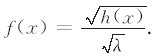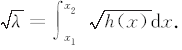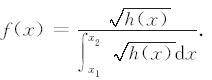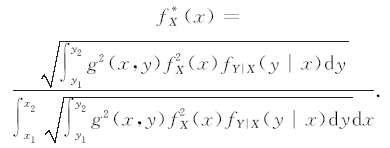2.2 将最优偏倚抽样密度函数应用于多区域投篮问题

$g(x,y)=y.$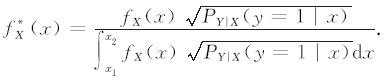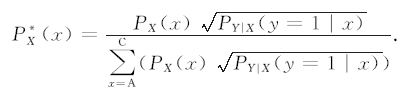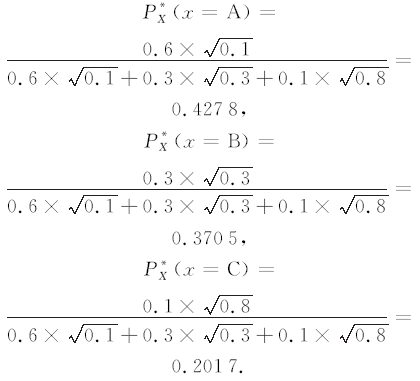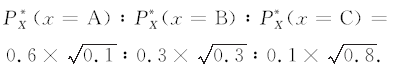$w(x)=PX(x)PX*(x).$表2 未采用偏倚抽样时投篮问题的均方差表3 采用最优偏倚抽样时投篮问题的均方差

 Figure Option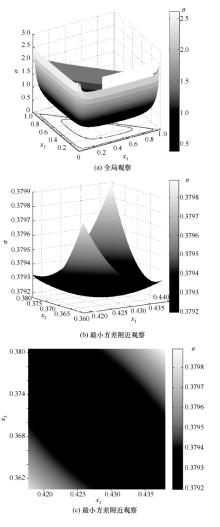图1 方差σ随分配比例x1、x2的变化趋势

3 源项最优偏倚抽样在实际粒子输运模拟中的实例分析

$p1(r)=e-μ(r-R1)=eμR1·e-μr=C1e-μr.$

$p2(r)=Ω计数区域4π=S球冠4πr2=2πrh4πr2=h2r=r02/r2r=C21r2,p(r)=p1(r)p2(r)=C3e-μrr2.$

$PY|R(y=1|r)=p(r)=C3e-μrr2.$

$fR(r)=3R23-R13r2, (R1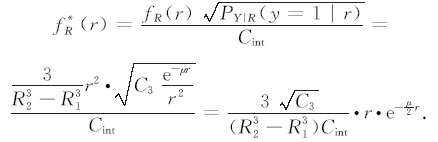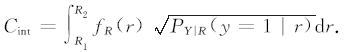3.1 用分层抽样的方法进行源项最优偏倚抽样

$fR*(r)∝r·e-μ2r$

$fi=ri·e-μ2ri.$

fi归一化即得到模拟粒子数份额,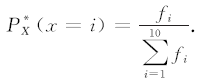$wi=viPX*(x=i).$表2 分层偏倚抽样参数

3.2 用连续的密度函数进行源项最优偏倚抽样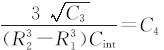, 根据 $∫R1R2fR*(r)dr=1可求出$

$C4=1∫R1R2r·e-μ2rdr=1∫2070r·e-0.43532rdr=0.6875.$

$fR*(r)=C4·r·e-μ2r.$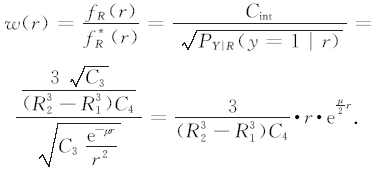3.3 计算结果比较表3 3种偏倚抽样类型模拟结果表3 3种偏倚抽样类型模拟结果

4 结 论

The authors have declared that no competing interests exist.

  裴鹿成. 蒙特卡罗方法中的若干问题[J]. 原子能科学技术, 1963(6): 422-431. PEI Lucheng. Several issues in Monte Carlo method[J]. Atomic Energy Science and Technology, 1963(6): 422-431. (in Chinese) [本文引用:1] [CJCR: 0.426]  董秀芳. 蒙特卡罗方法及其基本特点[J]. 原子能科学技术, 1978(3): 277-289. DONG Xiufang. Monte Carlo method and its basic characteristics[J]. Atomic Energy Science and Technology, 1978(3): 277-289. (in Chinese) [本文引用:1] [CJCR: 0.426]  朱辉, 刘义保, 游运. 蒙特卡罗方法与拟蒙特卡罗方法的历史、现状及展望[J]. 东华理工大学学报: 自然科学版, 2010, 33(4): 357-362. ZHU Hui, LIU Yibao, YOU Yun. Monte Carlo method and quasi-Monte Carlo method[J]. Journal of East China Institute of Technology: Natural Science, 2010, 33(4): 357-362. (in Chinese) [本文引用:1] [CJCR: 0.607]  裴鹿成, 张孝泽. 蒙特卡罗方法及其在粒子输运问题中的应用 [M]. 北京: 科学出版社, 1986. PEI Lucheng, ZHANG Xiaoze. Monte Carlo Methods and Application in Particle Transportation Problem [M]. Beijing: Science Press, 1986. (in Chinese) [本文引用:2]  裴鹿成. 计算机随机模拟 [M]. 长沙: 湖南科学出版社, 1989. PEI Lucheng. Computer Random Simulation [M]. Changsha: Hunan Science & Technology Press, 1989. (in Chinese) [本文引用:1]  朱永生. 实验物理中的概率和统计 [M]. 北京: 原子能出版社, 2006. ZHU Yongsheng. Probability and Statistics in Experimental Physics [M]. Beijing: Atomic Energy Press, 2006. (in Chinese) [本文引用:1]  张崭, 李君利, 武祯, 等. 内照射小器官剂量计算中减方差技巧的比较和应用[J]. 清华大学学报: 自然科学版, 2007, 47(S1): 1051-1056. ZHANG Zhan, LI Junli, WU Zhen, et al. Comparison and application of variance-reduction techniques used in internal radiation dose calculations for small organs[J]. Journal of Tsinghua University: Science and Technology, 2007, 47(S1): 1051-1056. (in Chinese) [本文引用:2] [CJCR: 0.609]  武祯, 李君利. 用于探测器校正因子计算的Monte Carlo方法[J]. 清华大学学报: 自然科学版, 2006, 46(9): 1585-1588. WU Zhen, LI Junli. Monte Carlo method for calculating particle radiation detector correction factors[J]. Journal of Tsinghua University: Science and Technology, 2006, 46(9): 1585-1588. (in Chinese) [本文引用:2] [CJCR: 0.609]  Bielajew A F. Correction factors for thick-walled ionization chambers in point-source photon beams[J]. Phys Med Biol, 1990, 35(4): 501-516. [本文引用:1] [JCR: 2.922]  Hedtjarn H, Carlsson G, Williamson J F. Accelerated Monte Carlo-based dose calculations for brachytherapy planning using correlated sampling[J]. Phys Med Biol, 2002, 47(3): 351-376. [本文引用:1] [JCR: 2.922]  Buckley L A, Kawrakow I, Rogers D W O. CSnrc: Correlated sampling Monte Carlo calculations using EGSnrc[J]. Med Phys, 2004, 31(12): 3425-3435. [本文引用:1] [JCR: 3.012]  武祯, 李君利, 程建平. 一种改进的计算探测器校正因子的相关抽样方法[J]. 高能物理与核物理, 2006, 30(8): 771-775. WU Zhen, LI Junli, CHENG Jianping. An improved correlated sampling method for calculating correction factor of detector[J]. High Energy Physics and Nuclear Physics, 2006, 30(8): 771-775. (in Chinese) [本文引用:1] [JCR: 0.233] [CJCR: 0.281]  许淑艳. 蒙特卡罗方法在实验核物理中的应用 [M]. 北京: 原子能出版社, 1996. XU Shuyan. Monte Carlo Method in Experimental Nuclear Physics [M]. Beijing: Atomic Energy Press, 1996. (in Chinese) [本文引用:1]  邱睿, 李君利, 曾志. 邮件辐照系统屏蔽的Monte Carlo计算[J]. 清华大学学报: 自然科学版, 2004, 44(3): 297-300. QIU Rui, LI Junli, ZENG Zhi. Monte Carlo calculation of the shielding of mail irradiation systems[J]. Journal of Tsinghua University: Science and Technology, 2004, 44(3): 297-300. (in Chinese) [本文引用:1] [CJCR: 0.609]  王汝赡, 姜宏宇. 方向偏移法及其在光子输运模拟中的应用[J]. 核电子学与探测技术, 1998, 18(3): 177-181. WANG Rushan, JIANG Hongyu. Direction biasing method and its application in γ ray transportation simulation[J]. Nuctear Eleetronics & Detection Technology, 1998, 18(3): 177-181. (in Chinese) [本文引用:2]  Olsher R H. A practical look at Monte Carlo variance reduction methods in radiation shielding[J]. Nuclear Engineering and Technology, 2006, 38(4): 225-230. [本文引用:1] [JCR: 0.758]  MCNP Monte Carlo Team, X-5. MCNP5_RSICC_1. 30, LA-UR-04. 5921 [R]. Los Alamos, NM: Los Alamos National Laboratory, 2004. [本文引用:1]  Hendricks J S, McKinney G W, Waters L S, et al. MCNPX, Version 2. 5. 3, LA-UR-04-0569 [R]. Los Alamos, NM: Los Alamos National Laboratory, 2004. [本文引用:1]  Booth T E. A Sample Problem in Variance Reduction in MCNP, LA-10363-MS [R]. Los Alamos, NM: Los Alamos National Laboratory, 1985. [本文引用:1]  Fassò A, Ferrari A, Ranft J, et al. FLUKA: Performances and applications in the intermediate energy range [C]//Proc 1st AEN/NEA Specialists' Meeting on Shielding Aspects of Accelerators, Targets and Irradiation Facilities (SATIF 1). Arlington, TX: OECD Documents, 1995: 287-304. [本文引用:1]  Allison J, Amako K, Apostolakis J, et al. GEANT4 developments and applications[J]. IEEE Transactions on Nuclear Science, 2006, 53(1): 270-277. [本文引用:1] [JCR: 1.455]